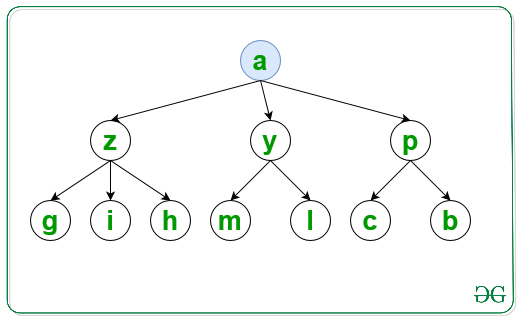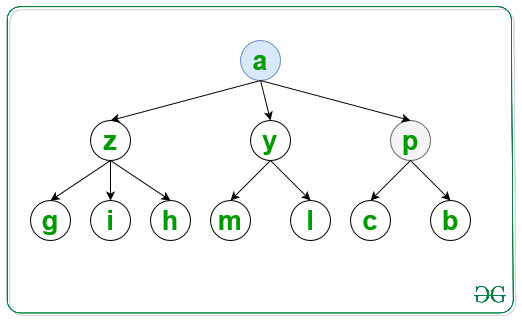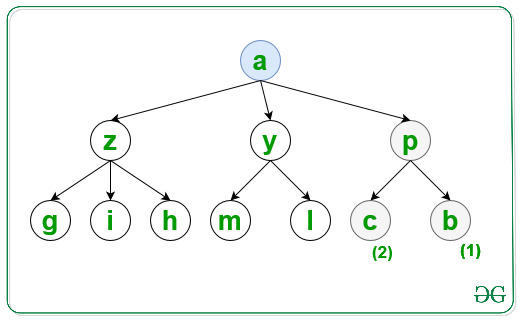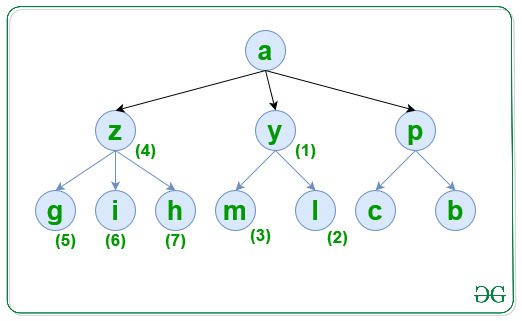# Traverse graph in lexicographical order of nodes using DFS

• Last Updated : 20 Jul, 2021

Given a graph, G consisting of N nodes, a source S, and an array Edges[] of type {u, v} that denotes that there is an undirected edge between node u and v, the task is to traverse the graph in lexicographical order using DFS.

Examples:

Input: N = 10, M = 10, S = ‘a’, Edges[] = { { ‘a’, ‘y’ }, { ‘a’, ‘z’ }, { ‘a’, ‘p’ }, { ‘p’, ‘c’ }, { ‘p’, ‘b’ }, { ‘y’, ‘m’ }, { ‘y’, ‘l’ }, { ‘z’, ‘h’ }, { ‘z’, ‘g’ }, { ‘z’, ‘i’ } }
Output: a p b c y l m z g h i

Explanation:
For the first level visit the node and print it:Similarly visited the second level node p which is lexicographical smallest as:Similarly visited the third level for node p in lexicographical order as:Now the final traversal is shown in the below image and labelled as increasing order of number:Input: N = 6, S = ‘a’, Edges[] = { { ‘a’, ‘e’ }, { ‘a’, ‘d’ }, { ‘e’, ‘b’ }, { ‘e’, ‘c’ }, { ‘d’, ‘f’ }, { ‘d’, ‘g’ } }
Output: a d f g e b c

Approach: Follow the steps below to solve the problem:

• Initialize a map, say G to store all the adjacent nodes of a node according to lexicographical order of the nodes.
• Initialize a map, say vis to check if a node is already traversed or not.
• Traverse the Edges[] array and store all the adjacent nodes of each node of the graph in G.
• Finally, traverse the graph using DFS and print the visited nodes of the graph.

Below is the implementation of the above approach:

## C++

 `// C++ program  for the above approach``#include ``using` `namespace` `std;` `// Function to traverse the graph in``// lexicographical order using DFS``void` `LexiDFS(map<``char``, set<``char``> >& G,``             ``char` `S, map<``char``, ``bool``>& vis)``{``    ``// Mark S as visited nodes``    ``vis[S] = ``true``;` `    ``// Print value of visited nodes``    ``cout << S << ``" "``;` `    ``// Traverse all adjacent nodes of S``    ``for` `(``auto` `i = G[S].begin();``         ``i != G[S].end(); i++) {` `        ``// If i is not visited``        ``if` `(!vis[*i]) {` `            ``// Traverse all the nodes``            ``// which is connected to i``            ``LexiDFS(G, *i, vis);``        ``}``    ``}``}` `// Utility Function to traverse graph``// in lexicographical order of nodes``void` `CreateGraph(``int` `N, ``int` `M, ``int` `S,``                 ``char` `Edges[])``{``    ``// Store all the adjacent nodes``    ``// of each node of a graph``    ``map<``char``, set<``char``> > G;` `    ``// Traverse Edges[] array``    ``for` `(``int` `i = 0; i < M; i++) {` `        ``// Add the edges``        ``G[Edges[i]].insert(``            ``Edges[i]);``    ``}` `    ``// Check if a node is already``    ``// visited or not``    ``map<``char``, ``bool``> vis;` `    ``// Function Call``    ``LexiDFS(G, S, vis);``}` `// Driver Code``int` `main()``{``    ``int` `N = 10, M = 10, S = ``'a'``;``    ``char` `Edges[M]``        ``= { { ``'a'``, ``'y'` `}, { ``'a'``, ``'z'` `},``            ``{ ``'a'``, ``'p'` `}, { ``'p'``, ``'c'` `},``            ``{ ``'p'``, ``'b'` `}, { ``'y'``, ``'m'` `},``            ``{ ``'y'``, ``'l'` `}, { ``'z'``, ``'h'` `},``            ``{ ``'z'``, ``'g'` `}, { ``'z'``, ``'i'` `} };` `    ``// Function Call``    ``CreateGraph(N, M, S, Edges);` `    ``return` `0;``}`

## Java

 `// Java program for above approach``import` `java.util.*;` `class` `Graph{` `// Function to traverse the graph in``// lexicographical order using DFS``static` `void` `LexiDFS(HashMap> G,``            ``char` `S, HashMap vis)``{``    ` `    ``// Mark S as visited nodes``    ``vis.put(S, ``true``);` `    ``// Print value of visited nodes``    ``System.out.print(S + ``" "``);` `    ``// Traverse all adjacent nodes of S``    ``if` `(G.containsKey(S))``    ``{``        ``for``(``char` `i : G.get(S))``        ``{``            ` `            ``// If i is not visited``            ``if` `(!vis.containsKey(i) || !vis.get(i))``            ``{``                ` `                ``// Traverse all the nodes``                ``// which is connected to i``                ``LexiDFS(G, i, vis);``            ``}``        ``}``    ``}``}` `// Utility Function to traverse graph``// in lexicographical order of nodes``static` `void` `CreateGraph(``int` `N, ``int` `M, ``char` `S,``                        ``char``[][] Edges)``{``    ` `    ``// Store all the adjacent nodes``    ``// of each node of a graph``    ``HashMap> G = ``new` `HashMap<>();` `    ``// Traverse Edges[] array``    ``for``(``int` `i = ``0``; i < M; i++)``    ``{``        ``if` `(G.containsKey(Edges[i][``0``]))``        ``{``            ``Set temp = G.get(Edges[i][``0``]);``            ``temp.add(Edges[i][``1``]);``            ``G.put(Edges[i][``0``], temp);``        ``}``        ``else``        ``{``            ``Set temp = ``new` `HashSet<>();``            ``temp.add(Edges[i][``1``]);``            ``G.put(Edges[i][``0``], temp);``        ``}``    ``}``    ` `    ``// Check if a node is already visited or not``    ``HashMap vis = ``new` `HashMap<>();` `    ``LexiDFS(G, S, vis);``}` `// Driver code``public` `static` `void` `main(String[] args)``{``    ``int` `N = ``10``, M = ``10``;``    ``char` `S = ``'a'``;` `    ``char``[][] Edges = { { ``'a'``, ``'y'` `}, { ``'a'``, ``'z'` `},``                       ``{ ``'a'``, ``'p'` `}, { ``'p'``, ``'c'` `},``                       ``{ ``'p'``, ``'b'` `}, { ``'y'``, ``'m'` `},``                       ``{ ``'y'``, ``'l'` `}, { ``'z'``, ``'h'` `},``                       ``{ ``'z'``, ``'g'` `}, { ``'z'``, ``'i'` `} };` `    ``// Function Call``    ``CreateGraph(N, M, S, Edges);``}``}` `// This code is contributed by hritikrommie`

## Python3

 `# Python3 program  for the above approach``G ``=` `[[] ``for` `i ``in` `range``(``300``)]``vis ``=` `[``0` `for` `i ``in` `range``(``300``)]` `# Function to traverse the graph in``# lexicographical order using DFS``def` `LexiDFS(S):``    ``global` `G, vis``    ` `    ``# Mark S as visited nodes``    ``vis[``ord``(S)] ``=` `1` `    ``# Prvalue of visited nodes``    ``print` `(S,end``=``" "``)` `    ``# Traverse all adjacent nodes of S``    ``for` `i ``in` `G[``ord``(S)]:``        ``# If i is not visited``        ``if` `(``not` `vis[i]):``            ``# Traverse all the nodes``            ``# which is connected to i``            ``LexiDFS(``chr``(i))` `# Utility Function to traverse graph``# in lexicographical order of nodes``def` `CreateGraph(N, M, S, Edges):``    ``global` `G``    ``# Store all the adjacent nodes``    ``# of each node of a graph` `    ``# Traverse Edges[] array``    ``for` `i ``in` `Edges:``        ``# Add the edges``        ``G[``ord``(i[``0``])].append(``ord``(i[``1``]))``        ``G[``ord``(i[``0``])] ``=` `sorted``(G[``ord``(i[``0``])])` `    ``# Function Call``    ``LexiDFS(S)` `# Driver Code``if` `__name__ ``=``=` `'__main__'``:``    ``N ``=` `10``    ``M ``=` `10``    ``S ``=` `'a'``    ``Edges``=``[ [``'a'``, ``'y'` `],[ ``'a'``, ``'z'` `],``           ``[ ``'a'``, ``'p'` `],[ ``'p'``, ``'c'` `],``           ``[ ``'p'``, ``'b'` `],[ ``'y'``, ``'m'` `],``           ``[ ``'y'``, ``'l'` `],[ ``'z'``, ``'h'` `],``           ``[ ``'z'``, ``'g'` `],[ ``'z'``, ``'i'` `] ]` `    ``# Function Call``    ``CreateGraph(N, M, S, Edges);` `# This code is contributed by mohitkumar29.`

## Javascript

 ``

Output:

`a p b c y l m z g h i`

Time Complexity: O(N * log(N))
Auxiliary Space: O(N)

My Personal Notes arrow_drop_up